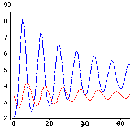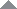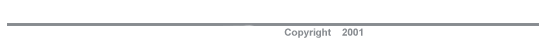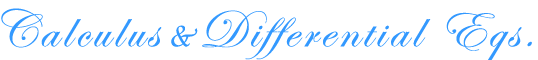Algebra Art Biology, Medicine & Psychology Business & Economics Calculus & Differential Equations Computer Science Data Analysis Discrete Math Engineering History & Geography Literature & Writing Math & Music Physical Sciences Pre-Algebra Statistics Trigonometry
 Case Studies in Calculus Case Studies in Calculus, a collection of worksheets promoting in depth exploration of selected applications of calculus, by Don Kreider and Dwight Lahr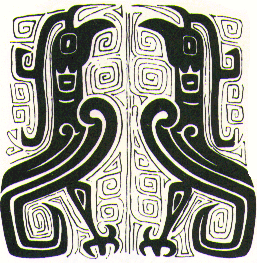Calcsite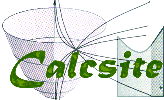Calcsite, a web site containing practice problems and tutorial videos, by Dwight Lahr and Dorothy Wallace Principles of Calculus Modeling Principles of Calculus Modeling, a slim volume to accompany the case studies, by Don Kreider and Dwight Lahr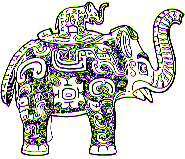Lake Victoria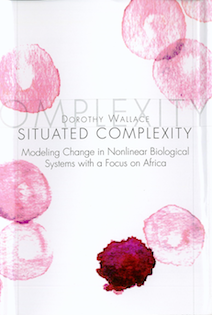Situated Complexity, Modeling Change in Nonlinear Biological Systems with a Focus on Africa, by Dorothy Wallace Water Hyacinth Distribution By Daniel Karnes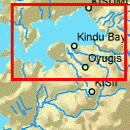Music and Computers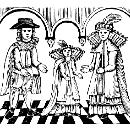Music and Computers, a web-based text on music and computers with many interactive Java applet examples, sound and graphic examples, by Phil Burk, Larry Polansky, Douglas Repetto, Mary Roberts, Dan Rockmore THE BELL THAT RINGS LIGHT THE BELL THAT RINGS LIGHT, A Primer in Quantum Mechanics and Chemical Bonding by Dorothy Wallace & Joseph J BelBruno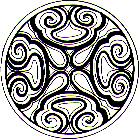Makin' Waves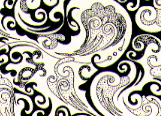Makin' Waves, an introduction to Fourier series and the wave equation, by Dorothy Wallace Integrated Math and Physical Science Text Integrated Math and Physical Science Text, containing a year of introductory physic and a year of calculus for students with advanced placement standing, by Marcia Groszek and Delo Mook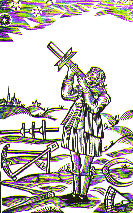That's Calculus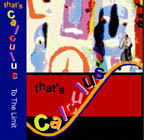That's Calculus, a video review of basic calculus concepts, by Edwin Gailits, Marcia Groszek and Dorothy Wallace Eyes on the Earth Eyes on the Earth, The mathematics of remote sensing, By Richard Birnie, Edwin Gailits and Dorothy Wallace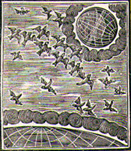An Introduction to Dynamical Systems And Mathematical Modelling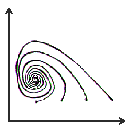An Introduction to Dynamical Systems and Mathematical Modelling, By Donal O'Shea Ordinary Differential Equation Machine The Big Green Ordinary Differential Equation Machine, a Java applet that integrates systems of ordinary differential equations, By B P Reid